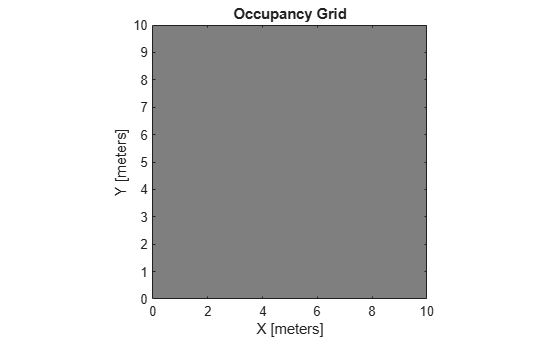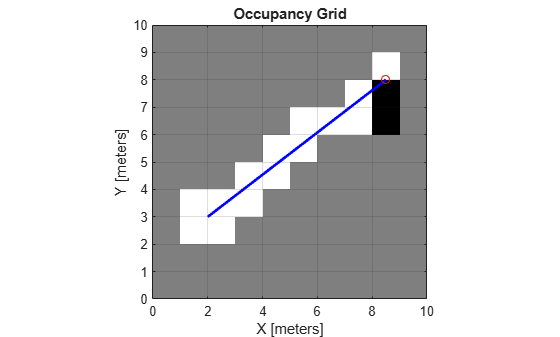# raycast

Compute cell indices along a ray

## Syntax

``````[endpoints,midpoints] = raycast(map,pose,range,angle)``````
``````[endpoints,midpoints] = raycast(map,p1,p2)``````

## Description

``````[endpoints,midpoints] = raycast(map,pose,range,angle)``` returns cell indices of the specified `map` for all cells traversed by a ray originating from the specified `pose` at the specified `angle` and `range` values. `endpoints` contains all indices touched by the end of the ray, with all other points included in `midpoints`.```

example

``````[endpoints,midpoints] = raycast(map,p1,p2)``` returns the cell indices of the line segment between the two specified points.```

## Examples

collapse all

Use the `raycast` method to generate cell indices for all cells traversed by a ray.

Create an empty map. A low-resolution map is used to illustrate the effected grid locations.

```map = occupancyMap(10,10,1); show(map)```Get the grid indices of the midpoints and end points of a ray from `[2 3]` to `[8.5 8]`. Set occupancy values for these grid indices. Midpoints are treated as open space. Update endpoints with an occupied observation.

```p1 = [2 3]; p2 = [8.5 8]; [endPts,midPts] = raycast(map,p1,p2); setOccupancy(map,midPts,zeros(length(midPts),1),'grid'); setOccupancy(map,endPts,ones(length(endPts),1),'grid');```

Plot the original ray over the map. Each grid cell touched by the line is updated. The starting point overlaps multiple cells, and the line touches the edge of certain cells, but all the cells are still updated.

```show(map) hold on plot([p1(1) p2(1)],[p1(2) p2(2)],'-b','LineWidth',2) plot(p2(1),p2(2),'or') grid on```## Input Arguments

collapse all

Map representation, specified as a `occupancyMap` object. This object represents the environment of the sensor. The object contains a matrix grid with values representing the probability of the occupancy of that cell. Values close to 1 represent a high probability that the cell contains an obstacle. Values close to 0 represent a high probability that the cell is not occupied and obstacle free.

Position and orientation of sensor, specified as an ```[x y theta]``` vector. The sensor pose is an x and y position with angular orientation theta (in radians) measured from the x-axis.

Range of ray, specified as a scalar in meters.

Angle of ray, specified as a scalar in radians. The angle value is for the corresponding `range`.

Starting point of ray, specified as an ```[x y]``` two-element vector. The point is defined in the world frame.

Endpoint of ray, specified as an ```[x y]``` two-element vector. The point is defined in the world frame.

## Output Arguments

collapse all

Endpoint indices, returned as an n-by-2 matrix of ```[i j]``` pairs, where n is the number of grid indices. The endpoints are where the `range` value hits at the specified `angle`. Multiple indices are returned when the endpoint lies on the boundary of multiple cells.

Midpoint indices, returned as an n-by-2 matrix of ```[i j]``` pairs, where n is the number of grid indices. This argument includes all grid indices the ray intersects, excluding the endpoint.